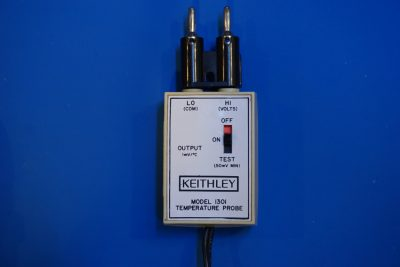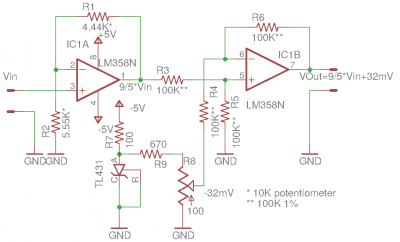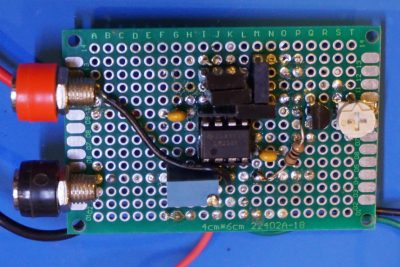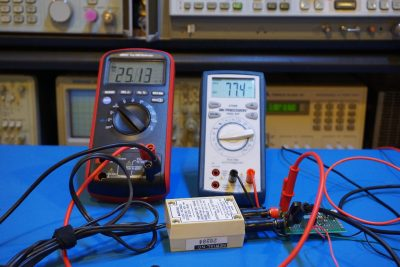I have an old Keithley 1301 temperature probe. This probe is an accessory to the Keithley 130A/131 multimeter and is capable of measuring temperatures between -55°C to 150 °C. Since it outputs an analog voltage linearly proportional to the temperature (1mV/°C), it can be used with any multimeter that has a mV range.The only issue is that the Keithley 1301 is for Celsius measurement only. To measure temperatures in Fahrenheit, we would need some kind circuitry that is capable of doing the following calculations:

$T_F = \frac{9}{5} T_c + 32$

One easy way to achieve this is to first convert the sensor output voltage to digital signals via an ADC and the calculation can be easily done using a microcontroller. The calculated result can either be displayed digitally (e.g. an LCD or a 7 segment display) or converted back to analog via a DAC.

Or we can do it entirely using only analog circuitry. The schematics below shows how this calculation is achieved. In this circuitry I used an LM358 dual op-amp. The first stage is configured as an non-inverting amplifier. Its gain is determined by the ratio between R1 and R2:

$Av=1+\frac{R1}{R2}$

To achieve the precise ratio, I used a 10K 10-turn trim pot so I could adjust R1/R2 ratio to be 4/5. This setting gives the non-inverting amplifier stage a gain of 9/5.

Now we needed to add on an offset (32mV) to the result so that the final output voltage would correspond to readings in Fahrenheit. This can be achieved in many ways. For example, we could use a simple non-inverting summing op-amp configuration and call it a day. While non-inverting summing op-amp seems to be the simplest, although it has many draw backs (e.g. output impedance changes in either channel can affect the gain accuracy of the other). Or we could use an inverting adder circuit and invert the result again with an inverting buffer. But this would require an additional op-amp. So I took another approach, using a differential amplifier configuration instead. In this configuration, four matched resistors (R3 through R6) are used. One draw back of this one op-amp differential amplifier is its limited input impedance. So to reduce the error caused by this, the output impedance of the sources to its inputs should be sufficiently low.

Because the differential amplifier amplifies the difference between the non-inverting and the inverting inputs into the op-amp, the offset voltage (in our case the 32mV voltage source) must first be negated. This can be easily achieved since we are using dual supply rails. As you can see in the circuit below, the TL431 is referenced to the ground and connected to the negative power rail via a 100 Ohm resistor. The -2.5V output is further divided via R9 and the 100Ω potentiometer to form the -32mV reference. The values chosen here ensures that the output impedance of the voltage source is significantly lower than the input impedance of the differential op-amp to minimize the summing error. Of course, you can always add a voltage follower between the voltage source and the differential input to further isolate the voltage source, but that would require an additional op-amp. Since our temperature sensor is only accurate to a few percentage points, our two op-amp circuit is more than adequate.This circuit is in essence how an analog computer works, albeit our calculations are limited to multiplication and subtraction only. Here is a picture of the circuit built on a protoboard:#### Calibration

This Celsius to Fahrenheit converter is calibrated in two stages. First we needed to calibrate the gain stage to have a gain of precicely 1.8. This is done using my EDC 216A voltage standard while adjusting the 10K potentiometer (R1, R2). The gain is then verified at a few spots between 0 and 100mV.

The second part is to set the -32mV reference. This is done by first shorting the input to the first op-amp (tie Vin to ground) and then adjusting potentiometer R8 so that the output the differential amplifier settles at 32mV.

The following picture shows the conversion result after the calibration. The voltage off the Keithley 1301 is 25.13 mV which corresponds to 25.13°C. The converter outputs 77.4 mV (77.4 °F) which is only less than .2 mV off the theoretical value.In the video below, you can me calibrating this analog Celsius to Fahrenheit converter and doing some measurements.